info@biomedres.us   +1 (502) 904-2126   One Westbrook Corporate Center, Suite 300, Westchester, IL 60154, USA   Site Map

Impact Factor : 0.548

# Kinetics Study The Catalyze Mode Of The Formamide Degradation Reaction By Aux Cluster (X=0, 1, 2, 3): Point, Line Or Surface? Volume 10 - Issue 5

Hongxia Liu* and Zizhong Liu

• Chemistry and Environment Science College, China

Received: October 29, 2018;   Published: November 09, 2018

*Corresponding author: Hongxia Liu, Chemistry and Environment Science College, China

DOI: 10.26717/BJSTR.2018.10.002024

## Abstract

The related energy and multi-channel CHONH2 degradation reaction potential energy surface under the catalysis of Aux (x=0, 1, 2, 3) cluster was studied, while its dynamic characterization has been investigated with density functional calculations. The geometries were fully optimized with the CCSD(T)//B3PW91 level. We can conclude two results:  The calculated results shown that the main pathway of the CHONH2 degradation reaction under the catalysis of Aux (x=0, 1, 2, 3) cluster can give the main product P1 (CO+NH3), while the minor product is P1(H2+HNCO) and P3 (H2O+HNC). We calculated the rate constant of the main reaction pathway, the calculated dynamic characterization indicating that the rate constants have the positive temperature dependence. According to the dynamic results and the energetically intermediates and transition states involved in the dominant paths, the reaction is expected to be occurred the most rapidly under the catalysis of Au2.  From the PESs, we can see the present invention, the singlet atom is the best catalysis and can catalyze the reaction better. The present invention studies may provide useful information on the issues of the reaction mechanism and product distributions.

Abbrevations: NBO: Natural Bond Orbital; ED: Electron Density; DFT: Density Functional Theory; PES: Potential Energy Surface; RECP: Relativistic Effective Core Potential; IRC: Intrinsic Reaction Coordinate; SCT: Small Curvature Tunnel Effect Correction

## Introduction

Gold has been enjoyed as the most inert metal for a very long time, until the pioneer of Haruta [1,2] found that the gold nanoparticles supported on some metal oxides showed surprisingly high catalytic activity for small molecule reaction at low temperature. , the Nano gold catalysis has picturesque growing in the field of heterogeneous catalysis [3-5]. Technologically, numerous potential industrialization applications of Nano gold catalysts were developed, such as synthesis of fine chemicals [6,7], selective hydrogenations [8,9], water gas shift reaction , carbon–carbon bond forming reaction , oxidation of organic compounds with molecular O2 [12,13], pollution and emission control  and fuel cell applications [15,16] Scientifically, a huge number of theoretical and experimental studies have been devoted to understand the wide gap between the chemical inertness of bulk gold and high catalytic activity of Nano gold[17-21]. The photolysis of formamide vapor has only been reported [22,23]. They studied the degradation reaction of formamide and gave the three major primary processes:According to their experimental conditions and results of the quantum yields ɸ, the threshold energy of channel a seems to be the lowest. Although the pyrolysis of formamide vapor apparently has not studied previously, several competing channels could also be expected in the thermal system. There are several reports about the competitive reactions in thermal systems [24,25], they conclude that the rate constants increased as the temperature arises. There are still unknown parameters in the descriptions and the rate constants, these two problems have not been entirely settled. Especially for complex bond-breaking reactions, the direct experimental determination of the threshold energy is almost impossible. Ab initio molecular orbital methods have developed rapidly and have provided heats of reaction, potential barriers, molecular geometries, and the vibrational frequencies of several intermediates and transition states. This valuable information can shed light on reaction mechanisms, and ab initio calculations have been applied to several unimolecular reactions. For complex bond-breaking reactions, information about the transition states is required to test the experimental results.

In the past two decades, the power of computational hardware, software gradually increased and therefore theoretical calculations became more and more popular and important to investigate structures, stabilities and activities of catalysts. calculation relies only on the electron density, which significantly decreases the computational complexities. It becomes an increasingly powerful and useful tool to evaluate various systems, predict catalytic activities, and provide enough accuracy to be compared to experimental data . So far, DFT calculation was widely used to investigate the mechanism of gold catalysis and provide fundamental physical insights into gold catalysis [17,27-28]. For example, selfconsistent density functional calculations showing that an isolated Au10 cluster should be able to catalyze the CO oxidation reaction even below room temperature and to use calculations can analyze the origin of this effect and suggest that the extraordinary reactivity can be traced back to special reaction geometries available at small particles in combination with an enhanced ability of low coordinated gold atoms to interact with molecules from the surroundings [17,18]. Based on the molecular orbital analysis and the DFT calculations, we have discussed the redistribution of in various bonding and antibonding orbitals and the energy of hyper conjugative interaction stabilization energy (E(2)) which have been calculated by natural bond orbital (NBO) analysis using DFT method to give clear evidence of stabilization originating from the hyperconjugation of various intramolecular interactions. The calculations are valuable for providing a reliable insight into the molecular properties.

In this work, we will give a brief review of theoretical investigations on CHONH2 degradation reaction catalyzed by Aux (x=0, 1, 2, 3) cluster, since most of the reviews focused on experimental results and the reviews of theoretical work were relatively rare. No major products are given and there is no available information on product channels, product distributions, and the reaction mechanism in the experiment. Considering the potential importance and the rather limited information, we carry out a detailed theoretical study on the potential energy surface (PES) of the CHONH2 degradation reaction to

a) Provide the elaborated addition channels on the CHONH2 degradation reaction PESs;

b) Give a deep insight into the mechanism of CHONH2 degradation reactions;

c) Calculate the rate constant of the H-abstraction. We hope our work will provide some valuable fundamental insights into title reaction.

## Computational Details

All Au clusters and CHONH2 molecule calculations are carried out using the Gaussian 09 program packages . The geometries of all the stationaries are optimized using the hybrid density functional B3PW91 method . As is known, full electron calculations for Au atom consume too much time, so it is necessary to introduce the relativistic effective core potential (RECP) to describe the inner core electrons. The 5s25p65d106s1 outermost valence electrons of the Au atom are described through the Lanl2dz base set [32, 33]. In order to obtain more reliable energetic data, higher level single- point energy calculations are performed at the multi-coefficient correlation method based on quadratic configuration interaction with single and double excitations (CCSD(T)) [34-38] by using the B3PW91 optimized geometries. To confirm that the transition states connect designated intermediates or products, intrinsic reaction coordinate (IRC) calculation is carried out at the B3PW91 level. Moreover, the CCSD(T) single-point energies with ZPE corrections are used in the following discussions.

NBO method  is performed to study orbital interactions supported by Gaussian 09 program. The localized orbital interactions, involved in non-covalent interactions, are quantified using second order perturbation theory, in which the secondorder energy (E(2)) was used to measure the interaction strengths. We use the Arrhenius formula to calculate the rate constant. The rate constant of the rate-controlling step along the main reaction channel was calculated among the temperature range 200～2000 K, which considers the small curvature tunnel effect correction (SCT) [40,41].

## Results and Discussions

For our convenient discussion, the energy of reactants R is set to be zero for reference. By means of the transition states and their connected isomers or products, a schematic potential energy surface (PES) of the most relevant reaction pathways is described for the CHONH2 degradation reaction catalyzed by Aux (x=0, 1, 2, 3) cluster and the optimized structures of the important stationary points are plotted in (Figure 1).

Table 1: Calculated enthalpies of formation (DH/kcal mol-1) according to eqn (1) for the adsorption CHONH2+Aux(x=0, 1, 2, 3 and 8) compounds.Table 3A: The thermodynamic functions H and S at B3PW91 level and the rate constants of the CHONH2 → P1 (NH3+CO) channel in the 200-2000 K temperature range.## CHONH2 Degradation Reaction by Gold Cluster

### The Related Energy

Data on formation enthalpies constitute an excellent means to establish whether theoretically predicted phases are likely to be stable, and such data may serve as a guide to evaluate possible reaction routes. For the exploration of the thermodynamic feasibility of accessing these compounds from the elements (eq. (1)) we have also computed the total energies for CHONH2 + Aux (x=0, 1, 2, 3) in their ground state structures with full geometry optimization. The adsorption enthalpies for CHONH2 + Aux (x=0, 1, 2, 3) were calculated from the difference in the total energy which are summarized in Table 1. The results establish unambiguously that (eq. (1)) expresses endothermic process for CHONH2 + A ux (x=0, 1, 2, 3) reaction.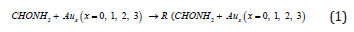The formation energy for the prototypical CHONH2 +Au1 are -71.4 and -63.5 kcal/mol respectively, indicating that CHONH2+Au1 is a thermodynamically stable phase at ambient conditions. This has already been established by a series of experimental and theoretical studies. Our estimated large positive values for the enthalpy of formation for the CHONH2+Au1 series also suggest that it might be possible to degradation compounds by singlet Au catalyst.

### Reaction Potential Energy Surface

A schematic potential energy surface of the CHONH2 degradation reaction obtained at the CCSD(T)//B3PW91 + ZPE level is plotted in Figure 1a. On the PES for the CHONH2 degradation reaction, 1,2-hydrogen-abstraction along with the cleavage of C-N bond can give product P1 (NH3+CO) via the transition state TSRP1. While CHONH2 also can degradation reaction by other two channels with the barrier TSRP2, TSRa and TSab. In view of the four barriers, it is impossible to overcome the barrier height for these four transition states which the energy barrier is too high. According to the PES in Figure 1a, there are two one-step and one two-steps degradation reaction channels which are the possible reaction pathways. From the kinetics, the pathway form P3 is more competitive. It needs to overcome the barrier height 71.7 kcal/mol and 40.9 kcal/mol lower than TSRP1 (80.0 kcal/mol) and TSRP2 (93.7 kcal/mol) about 8.3 kcal/mol and 22.0 kcal/mol. Meanwhile, the channel of forming P1 is one-step reaction pathway which is more feasible than two-steps reaction pathway. While from the thermodynamics, the energy of product P1 (5.4 kcal/mol) is lower than P2 and P3 by 10.2 kcal/ mol and 25.4 kcal/mol, respectively. Obviously, the formation of P1 is more favorable whatever in kinetics and in thermodynamics. The degradation reaction channel can be described as:While from the real condition, the most feasible reaction channel is hard to occur and degrade. How to degrade formamide in industrial wastewater has been one of the problems for environmental workers. In this work, we used the Au cluster to catalysis the CHONH2 degradation reaction. Seen from (Figure 1), and compared to the CHONH2 degradation reaction, the CHONH2+ Aux (x=0, 1, 2, 3) reaction mechanism is the same and the formation of P1 is all more favorable whatever in kinetics and in thermodynamics. The energy barrier of the transition state TSRP1 is 80.0 kcal/mol, 31.6 kcal/mol, 23.9 kcal/mol and 55.0 kcal/mol respectively which catalyzed by Aux (x=0, 1, 2, 3). From a kinetic perspective, the barrier of degradation reaction is the lowest when x =2. On the other hand, from the thermodynamic perspective, the degradation reaction can give off the most heat when x=1. It is shown that the energy variation of the transition state is consistent with the DE variation. However, because the inconsistency between kinetic and thermodynamic conclusions, it is difficult to determine which is the probable value of x in the formamide degradation reaction catalyzed by Aux (x=0, 1, 2, 3) at different temperature solely based on energies. To provide the exact x value of formamide degradation reaction catalyzed by Aux (x=0, 1, 2, 3), there is need to perform kinetic calculations.

Figure 1: A schematic potential energy surface of the CHONH2 degradation reaction obtained at the CCSD(T)//B3PW91 + ZPE level.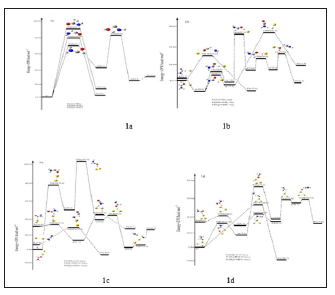In summary, starting from Reactant N H2CHO, we have found three possible reaction channels (Paths 1～3). All the intermediates and transition states of paths (Paths 1～3) proceeding lie above the reactant R at the CCSD(T)//B3PW91 level, therefore, (Paths 1～3) leading to P1 (NH3+CO), P2 (f + HNCO), and P3 (HNC + H2O) are much less competitive at normal temperature. Of these three unfeasible paths, we used the Au cluster to catalysis the CHONH2 degradation reaction. We found Path 1 which forms product P1 (NH3+CO) is all more favorable whatever in kinetics and in thermodynamics. The relative energy of TSRP1 which catalyzed by Aux (x=0, 1, 2, and 3) cluster lies 80.0 kcal/mol, 31.6 kcal/mol, 30.9 kcal/mol, and 55.0 kcal/mol; while thermodynamically, the formation of P1 is more favorable by 5.4 kcal/mol, -23.8 kcal/mol, -8.5 kcal/mol, and -20.7 kcal/mol. Thus, we predict that the actual yield of the product P1 may depend on the catalyst in the experiment. Therefore, as reflected in the final product distributions, we predict that (1) a total of three kinds of products P1 (NH3+CO), P2 (H2+HNCO), and P3 (HNC + H2O) should be observed; (2) the major product should be P1 (NH3+CO), while P2 (H2+HNCO) and P3 (HNC + H2O) are the less competitive products; (3) from the thermodynamic perspective, the degradation reaction can give off the most heat when x=1; (4) from a kinetic perspective, the barrier of degradation reaction is the most lowest when the x is equal to 2. Since there is lack of product information in experiment, we hope our present calculation may represent a useful model for understanding the mechanism and provide valuable information for further identification of the product distributions for the title reaction, which is experimentally unknown.

### NBO Analysis

The natural bond orbital (NBO) were calculated in order to understand various second-order interactions between the filled orbital of one subsystem and vacant orbital of another subsystem, which is a measure of the intermolecular delocalization or hyperconjugation. NBO analysis provides the most accurate possible “natural Lewis structure” picture of “j” because all orbital details are mathematically chosen to include the highest possible percentage of the electron density. A useful aspect of the NBO method is that it gives information about interactions of both filled and virtual orbital spaces that could enhance the analysis of intra-inter molecular interactions. The second-order Fock-matrix was carried out to evaluate the donor acceptor interactions in the NBO basis. The interactions result in a loss of occupancy from the localized NBO of the idealized Lewis structure into an empty non- Lewis orbital. For each donor (i) and acceptor (j) the stabilization energy (E2) associated with the delocalization i/j is determined as: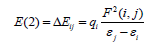where qi denotes the occupancy of donor orbital, F(i, j)represents the off-diagonal NBO Fock matrix element and ℇi and ℇj are orbital energies (diagonal elements), respectively. In NBO analysis large E(2) value shows the intensive interaction between electron donors and electron-acceptors and greater the extent of conjugation of the whole system, the possible intensive interactions are given in Table 2. The second-order perturbation theory analysis of Fock matrix in NBO basis shows strong intramolecular hyper-conjugative interactions of p electrons.

Table 2A: Second order perturbation theory analysis of Fock matrix in NBO basis of CHONH2a E(2) means energy of hyper conjugative interaction (stabilization energy).

b Energy difference between donor and acceptor i and j NBO orbitals.

c F(i, j) is the fork matrix element between i and j NBO orbitals.

Table 2B: Second order perturbation theory analysis of Fock matrix in NBO basis of CHONH2+Au1.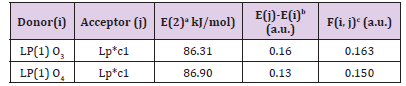a E(2) means energy of hyper conjugative interaction (stabilization energy).

b Energy difference between donor and acceptor i and j NBO orbitals.

c F(i, j) is the fork matrix element between i and j NBO orbitals.

Table 2C: Second order perturbation theory analysis of Fock matrix in NBO basis of CHONH2+Au2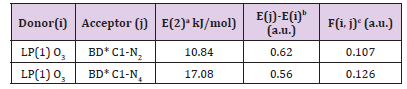a E(2) means energy of hyper conjugative interaction (stabilization energy).

b Energy difference between donor and acceptor i and j NBO orbitals.

c F(i, j) is the fork matrix element between i and j NBO orbitals.

Table 2D: Second order perturbation theory analysis of Fock matrix in NBO basis of CHONH2+Au3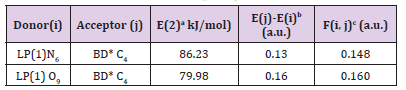a E(2) means energy of hyper conjugative interaction (stabilization energy).

b Energy difference between donor and acceptor i and j NBO orbitals.

c F(i, j) is the fork matrix element between i and j NBO orbitals.

In Table 2, the perturbation energies of significant donor acceptor interactions are present. (1) In CHONH2 , the interactions between the LP (1) N4 and the anti-bonding of C1-O3 have the highest E(2) value around 60.14 kJ/mol; (2) In CHONH2 +Au1, the interactions between the LP (1) N4 and the anti-bonding of C1 have the highest E(2) value around 86.90 kJ/mol. The other significant interactions giving stronger stabilization energy value of 86.31 kJ/ mol to the structure are the interactions between LP (1) O3 and LP* (1) C1; (3) In CHONH2 +Au2, the interactions between the LP (2) O3 and the anti-bonding of C1–N4 have the highest E(2) value around 17.08 kJ/mol; (4) In CHONH2 +Au3, the interactions between the LP (1) N6 and the anti-bonding of C4 have the highest E(2) value around 86.23 kJ/mol. The other significant interactions giving stronger stabilization energy value of 79.98 kJ/mol to the structure are the interactions between LP (3) O9 and LP* (1) C4. Simultaneously, substantial overlaps (Figure 2) of interaction orbitals are reduced along with the decrements of E(2)s. The intermolecular electron transfer occurs from the lone pairs of oxygen atom to the antibonding orbitals of C–N bond, which can well explain the breaking of C–N bond easily. Comparing with C–H and N–H bonds et.al, partial orbital interactions between C and N in CHONH2 +Au2 is weaker. Overall, the E(2)s in Table 2 indicates that the C–N bond in CHONH2+Au2 is weaker than those in CHONH2 + Aux (x=0, 1, 3) to some degree. This result is well consistent with kinetic parameters obtained among reaction Path 1 in above reaction potential energy surface analyses (Figure 3).

Figure 2: Substantial overlaps of interaction orbitals of the CHONH2+Aux(x=0, 1, 2, 3)→ P1 (NH3+CO+Aux(x=0, 1, 2, 3))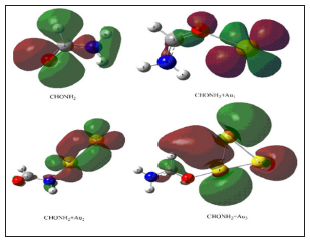Figure 3: The rate constant of the CHONH2+Aux(x=0, 1, 2, 3)→ P1 (NH3+CO+Aux(x=0, 1, 2, 3)) at the temperature of 298 K.Kinetics: In this section, we performed the dynamic calculation for the degradation reaction CHONH2 + A ux (x=0, 1, 2, 3). The calculation of the thermodynamic functions H and S at B3PW91 level was used for obtaining the rate constant results of the dominant channel in the 200～2000 K temperature range. Results are listed in Table 3. Rate constants were calculated as follows:Where the type of ΔrHm and ΔrSm are respectively for the standard molar activation enthalpy and entropy of reaction system. A is the frequency factor, and kb is Boltzmann constant. It is seen from (Table 3), it shows that the rate constant k of this reaction increases significantly as the temperature increase. Among the range of 200～2000 K, we find that the theoretical rate constants are slightly positive temperature dependence at 200～2000 K. So, it is favorable to the most probable reaction at higher temperature. Seen from Fig.3, compared among these three kinds of reactions, the rate constant of CHONH2 +Au2 is the biggest one which is suitable with the kinetic analysis of the PESs at the temperature of 298 K. Therefore, we hope our present calculation may represent a useful model to understand the mechanism and provide valuable information for the title reaction, and to deeply understand the mechanism of the title reaction.

Table 3B: The thermodynamic functions H and S at B3PW91 level and the rate constants of the CHONH2+Au1→ P1 (NH3+CO+Au1) channel in the 200-2000 K temperature range.Table 3C: OThe thermodynamic functions H and S at B3PW91 level and the rate constants of the CHONH2+Au2→ P1 (NH3+CO+Au2) channel in the 200-2000 K temperature range.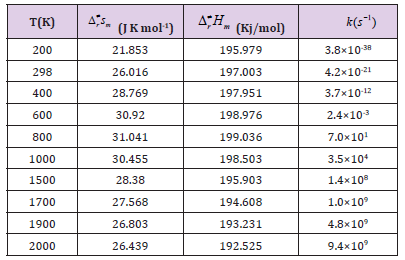Table 3D: The thermodynamic functions H and S at B3PW91 level and the rate constants of the CHONH2+Au3→ P1 (NH3+CO+Au3) channel in the 200-2000 K temperature range.## Conclusion

We have presented a detailed investigation on the related energy and multi-channel CHONH2 degradation reaction potential energy surface under the catalysis of Aux (x=0, 1, 2, 3) cluster and its dynamic characterization using a detailed quantum chemical methods. The following important conclusions are obtained

a) The prediction of CHONH2 degradation reaction under the catalysis of Aux (x=0, 1, 2, 3) cluster with large positive formation enthalpy hopefully will inspire and guide catalysis efforts in this direction. NBO theory is applied to characterize the nature of the intermolecular orbital interactions in the CHONH2 + A ux (x=0, 1, 2, 3). The calculation show that the maximum formation energy and thermodynamics prove that the CHONH2 + Au1 reaction is the most possible, while kinetic and NBO analyses prove that reaction CHONH2 + Au2 is the most plausible. In summary, the rate constant of CHONH2 +Au2 is the biggest one which is suitable with the kinetic and NBO analysis.

b) The multi-channel CHONH2 degradation reaction potential energy surface under the catalysis of Aux (x=0, 1, 2, 3) cluster is performed to explore the reaction mechanism. Our calculation show that one primary channel is obtained which the H-shift reaction can give the main product P1 (NH3+CO+ Aux (x=0, 1, 2, 3)). And CHONH2 + Aux (x=0, 1, 2, 3) reaction occurs mainly in the high-temperature range which plays a more important role as the temperature increase. The present theoretical studies may provide useful information on the reaction mechanism.

c) The dynamic characterization show that the theoretical rate constants are slightly positive temperature dependence at 200～2000 K. The rate constant k of this reaction increases significantly as the temperature increase. The analyses consistently support the notion that the formamide degradation reaction can be catalyzed by singlet atom whatever point, line and surface in catalysts. Until now, there are no experimental studies available to verify our results, but we hope to motivate experimentalists to measure the rate constant.

## Acknowledgment

The project was supported by the Higher educational Scientific research projects of Inner Mongolia (NJZY030), the Natural Science Foundation of Inner Mongolia (2012MS0218), the Talent Development Foundation of Inner Mongolia, the Inner Mongolia Normal University Research Fund Project (KYZR1119, 2017ZRZD001), the Natural Science Foundation of Inner Mongolia (2014BS0206), and the Scientific research project of the Inner Mongolia colleges and Universities (NJZY030).